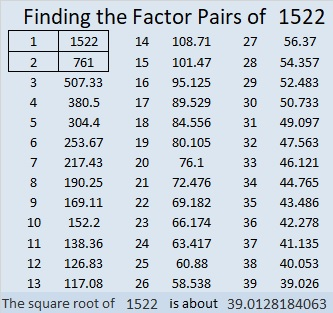# 1522 Happy Birthday, Sue, in Spite of All the Chaos!

Contents

### Today’s Puzzle:

Today is my sister’s birthday. This year has been a turbulent and chaotic year for many people including her. A hurricane completely damaged her home this summer. Sue, here’s hoping that the coming year will be much brighter for you. Here is a chaotic-looking puzzle for your birthday. If you find all the products after you find all the factors, it will look a lot more orderly. Have a very happy birthday today!### Factors of 1522:

• 1522 is a composite number.
• Prime factorization: 1522 = 2 × 761.
• 1522 has no exponents greater than 1 in its prime factorization, so √1522 cannot be simplified.
• The exponents in the prime factorization are 1, and 1. Adding one to each exponent and multiplying we get (1 + 1)(1 + 1) = 2 × 2 = 4. Therefore 1522 has exactly 4 factors.
• The factors of 1522 are outlined with their factor pair partners in the graphic below.### More Facts about the Number 1522:

1522 is the sum of two squares:
39² + 1² = 1522.

1522 is the hypotenuse of a Pythagorean triple:
78-1520-1522 calculated from 2(39)(1), 39² – 1² , 39² + 1².

From OEIS.org we learn this curious fact:
The digits of 1522 are 1, 5, 2, and 2.
The squares of each of those digits are 1, 25, 4, and 4.
12544 is a perfect square. It is 112².

This site uses Akismet to reduce spam. Learn how your comment data is processed.